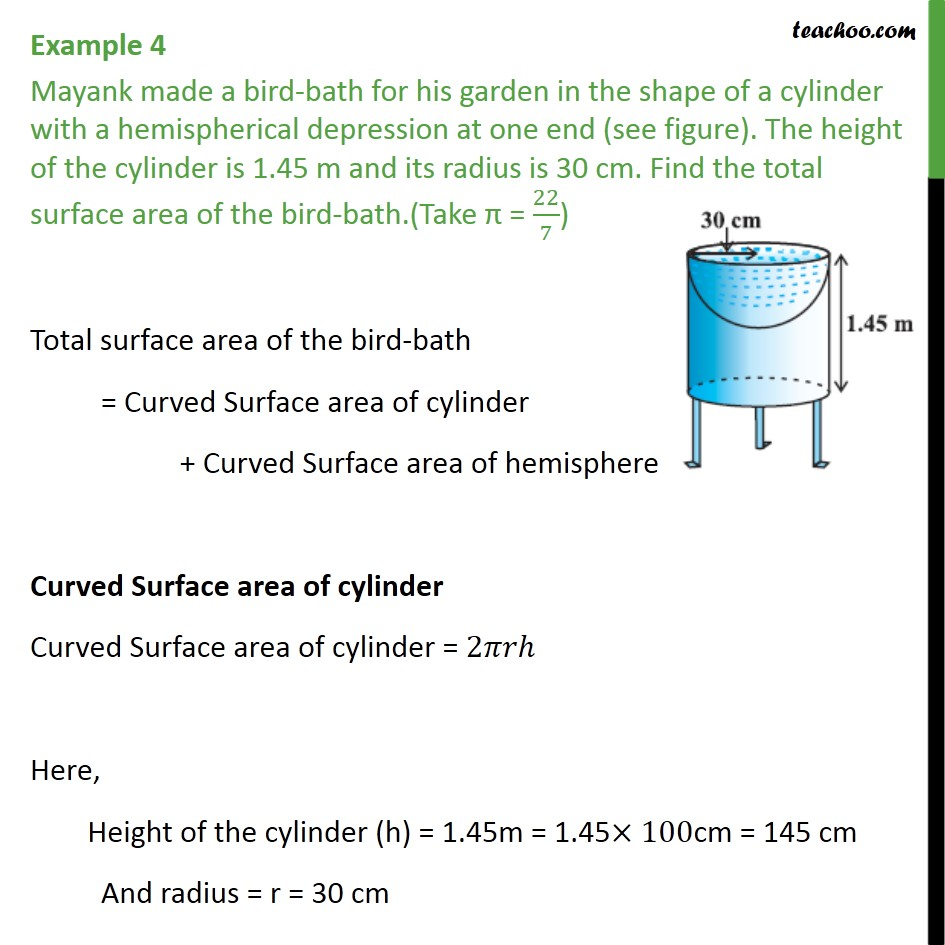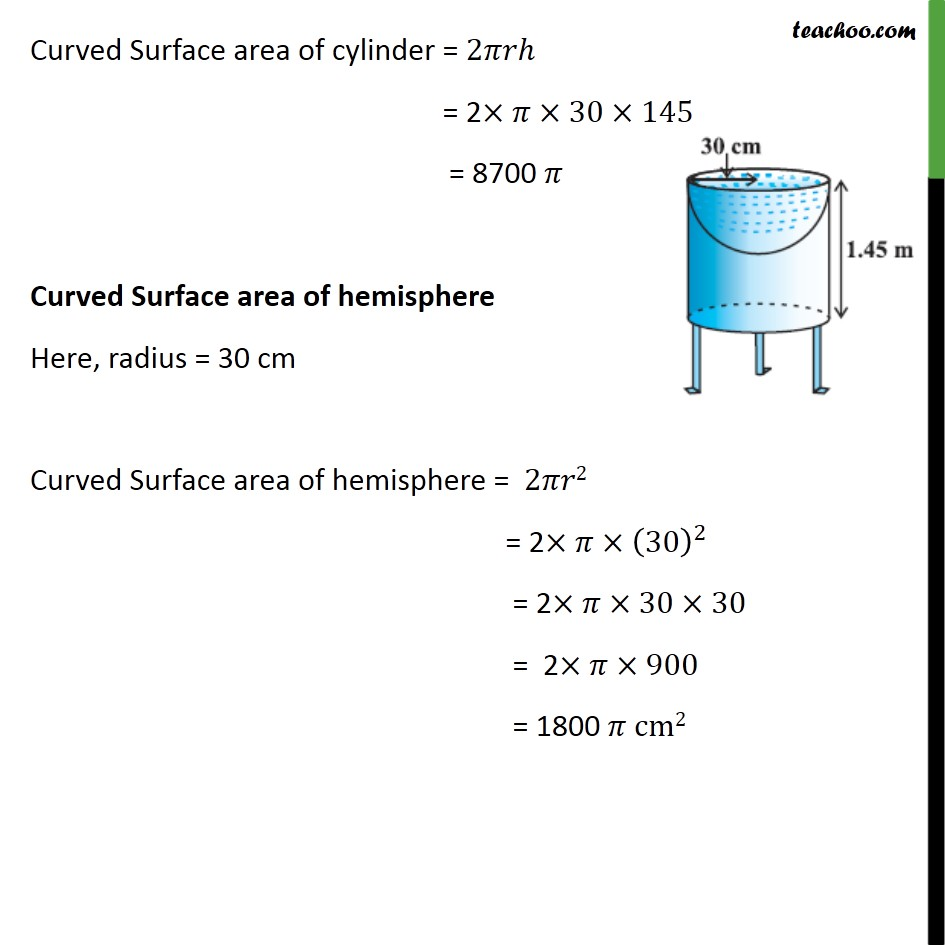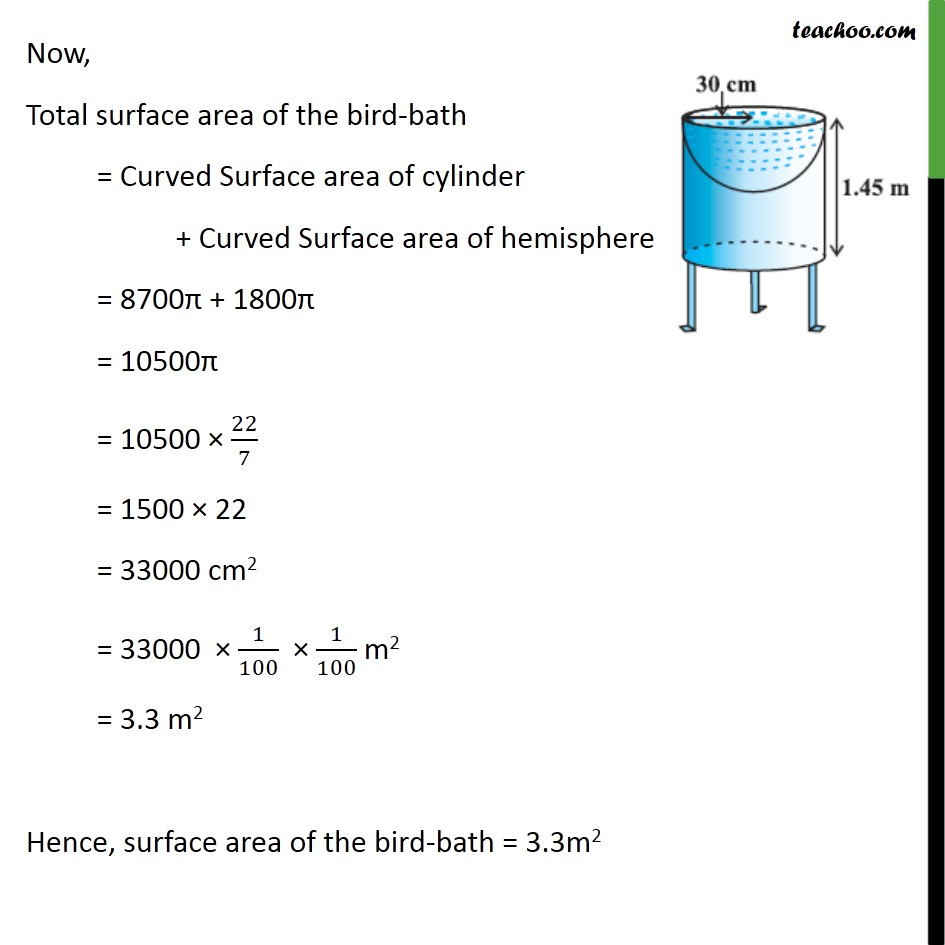Subscribe to our Youtube Channel - https://you.tube/teachoo

1. Chapter 13 Class 10 Surface Areas and Volumes
2. Concept wise
3. Surface Area - Subtracted

Transcript

Example 4 Mayank made a bird-bath for his garden in the shape of a cylinder with a hemispherical depression at one end (see figure). The height of the cylinder is 1.45 m and its radius is 30 cm. Find the total surface area of the bird-bath.(Take = 22/7) Total surface area of the bird-bath = Curved Surface area of cylinder + Curved Surface area of hemisphere Curved Surface area of cylinder Curved Surface area of cylinder = 2 Here, Height of the cylinder (h) = 1.45m = 1.45 100cm = 145 cm And radius = r = 30 cm Curved Surface area of cylinder = 2 = 2 30 145 = 8700 Curved Surface area of hemisphere Here, radius = 30 cm Curved Surface area of hemisphere = 2 2 = 2 (30)^2 = 2 30 30 = 2 900 = 1800 cm2 Now, Total surface area of the bird-bath = Curved Surface area of cylinder + Curved Surface area of hemisphere = 8700 + 1800 = 10500 = 10500 22/7 = 1500 22 = 33000 cm2 = 33000 1/100 1/100 m2 = 3.3 m2 Hence, surface area of the bird-bath = 3.3m2

Surface Area - Subtracted